## Plus or Minus

The symbol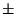is used to denote a quantity which should be both added and subtracted, as in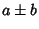. The symbol can be used to denote a range of uncertainty, or to denote a pair of quantities, such as the roots given by the Quadratic FormulaWhen order is relevant, the symbolis also used, so an expression of the form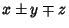is interpreted asor. In contrast, the expression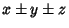is interpreted to mean the set of four quantities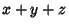,,, and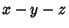.